### Vakil

Posted on September 15, 2018

Here are my exercise solutions and notes for the beginning of Vakil’s Foundations of Algebraic Geometry.

### Chapter 1: Category Theory

1.2.A.

1. The set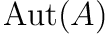in a groupoid with one object is a group, and vice versa.

2. Pick any groupoid with two or more objects?

1.2.B. Identity and associativity under the operation of composition follows from the definition of morphisms in a category. Thus the set of invertible automorphisms forms a group.

The automorphism groups in the category of sets are the symmetric groups. Assuming vector spaces are finite dimensional, their automorphism groups are general linear groups. For infinite dimensional vector spaces more complicated groups would arise.

If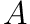is isomorphic to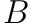thenis isomorphic to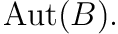Suppose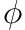is an isomorphism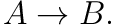Let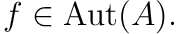Observe that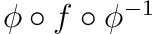is a morphism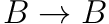with inverse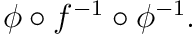So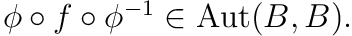Let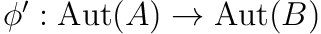be defined by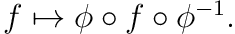Note that this map is surjective by the argument above. Moreover, for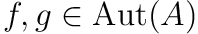, observe that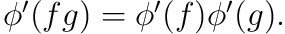Thus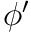is a homomorphism. Finally, suppose that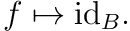ThenThushas trivial kernel and is hence injective.

The point of the “isomorphic, but not canonically isomorphic” comment is that although the automorphism groups are pairwise isomorphic, there is not necessarily a “canonical” way of identifying the groups; that is, there might be distinct isomorphisms between the same two automorphism groups. This is classically the case with pointed fundamental groups in the situation that Vakill describes.

1.3.A. Let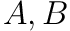be two initial objects. Let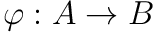,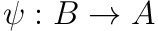be the only morphisms. Observe that both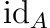and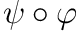are morphisms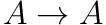, thus they must be equal sinceis initial. Similarly for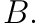Thus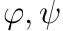are isomorphisms.

A similar argument follows forfinal.

1.3.B. In Sets and Top the initial object is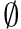and the final object is a singleton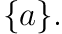In Rings the initial object is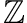and the final object is the zero ring.

1.3.C. Let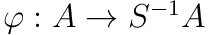be the natural homomorphism. Observe that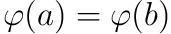if and only if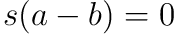for some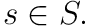Thus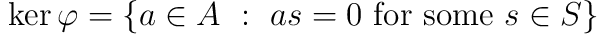, and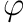injective if and only if there exist no zero divisors in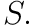1.3.D. Suppose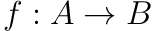is an-algebra homomorphism sending each element of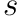to a unit inObserve that if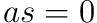for some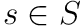, then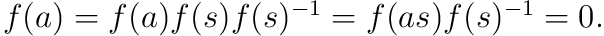Thus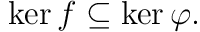Hence there exists a unique map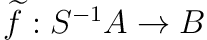such that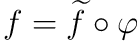(see Lemma 1.2 in my [Fulton notes][BroFulton]).

1.3.E. Clearly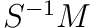as defined in the hint is an abelian group under the defined addition, with identity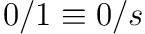for allMoreover, the defined ring action defined satisfies the axioms forto be an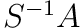-module.

1.3.F.

(a) Define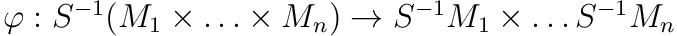by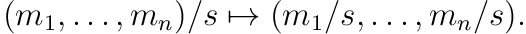Observe thatThereforeis a group homomorphism, and thus clearly an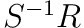-module homomorphism.

Suppose that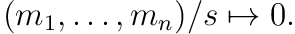Then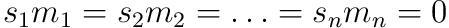for some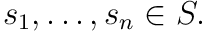Therefore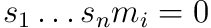for all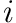and thus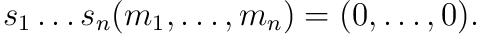Hence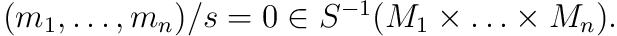Thus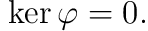Let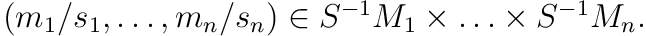Let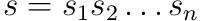and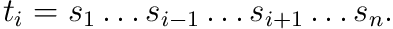Then observe that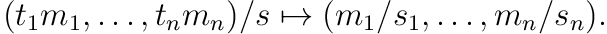Thusis surjective.

(b) The same argument above works equivalently for arbitrary direct sums.

(c) Let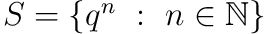for some prime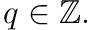Then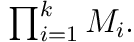See the corresponding exercise in my solutions to Fulton’s algebraic curve.